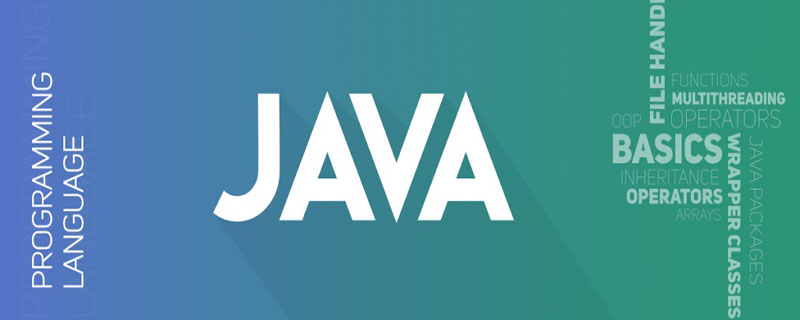# java高频率基础面试题——（九）_编程技术_编程开发技术教程

（更多相关面试题推荐：java面试题及答案

``` student(sno,sname,sage,ssex)学生表
course(cno,cname,tno) 课程表
sc(sno,cno,score) 成绩表
teacher(tno,tname) 教师表```

101，查询课程1的成绩比课程2的成绩高的所有学生的学号

```select a.sno from
(select sno,score from sc where cno=1) a,
(select sno,score from sc where cno=2) b
where a.score>b.score and a.sno=b.sno```

102，查询平均成绩大于60分的同学的学号和平均成绩

```select a.sno as "学号", avg(a.score) as "平均成绩"
from
(select sno,score from sc) a
group by sno having avg(a.score)>60```

103，查询所有同学的学号、姓名、选课数、总成绩

```select a.sno as 学号, b.sname as 姓名,
count(a.cno) as 选课数, sum(a.score) as 总成绩
from sc a, student b
where a.sno = b.sno
group by a.sno, b.sname```

```selectstudent.sno as 学号, student.sname as 姓名,
count(sc.cno) as 选课数, sum(score) as 总成绩
from student left Outer join sc on student.sno = sc.sno
group by student.sno, sname```

104，查询姓“张”的老师的个数

`selectcount(distinct(tname)) from teacher where tname like '张%‘`

```select tname as "姓名", count(distinct(tname)) as "人数"
from teacher
where tname like'张%'
group by tname```

105，查询没学过“张三”老师课的同学的学号、姓名

```select student.sno,student.sname from student
where sno not in (select distinct(sc.sno) from sc,course,teacher
where sc.cno=course.cno and teacher.tno=course.tno and teacher.tname='张三')```

（推荐学习java课程

106，查询同时学过课程1和课程2的同学的学号、姓名

```select sno, sname from student
where sno in (select sno from sc where sc.cno = 1)
and sno in (select sno from sc where sc.cno = 2)```

```selectc.sno, c.sname from
(select sno from sc where sc.cno = 1) a,
(select sno from sc where sc.cno = 2) b,
student c
where a.sno = b.sno and a.sno = c.sno```

```select student.sno,student.sname from student,sc where student.sno=sc.sno and sc.cno=1
and exists( select * from sc as sc_2 where sc_2.sno=sc.sno and sc_2.cno=2)```

107，查询学过“李四”老师所教所有课程的所有同学的学号、姓名

```select a.sno, a.sname from student a, sc b
where a.sno = b.sno and b.cno in
(select c.cno from course c, teacher d where c.tno = d.tno and d.tname = '李四')```

```select a.sno, a.sname from student a, sc b,
(select c.cno from course c, teacher d where c.tno = d.tno and d.tname = '李四') e
where a.sno = b.sno and b.cno = e.cno```

108，查询课程编号1的成绩比课程编号2的成绩高的所有同学的学号、姓名

```select a.sno, a.sname from student a,
(select sno, score from sc where cno = 1) b,
(select sno, score from sc where cno = 2) c
where b.score > c.score and b.sno = c.sno and a.sno = b.sno```

109，查询所有课程成绩小于60分的同学的学号、姓名

```select sno,sname from student
where sno not in (select distinct sno from sc where score > 60)```

110，查询至少有一门课程与学号为1的同学所学课程相同的同学的学号和姓名

```select distinct a.sno, a.sname
from student a, sc b
where a.sno <> 1 and a.sno=b.sno and
b.cno in (select cno from sc where sno = 1)```

```select s.sno,s.sname
from student s,
(select sc.sno
from sc
where sc.cno in (select sc1.cno from sc sc1 where sc1.sno=1)and sc.sno<>1
group by sc.sno)r1
where r1.sno=s.sno```

java高频率基础面试题——（九）

css中的“计算属性”是什么

## java基本数据类型各占多少字节_编程技术_亿码酷站

java基本数据类型各占多少字节_编程技术_亿码酷站

## java安装后怎么打开_编程技术_亿码酷站

java安装后怎么打开_编程技术_亿码酷站

## java与javascript的主要区别是什么

java与javascript的主要区别是什么